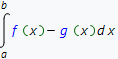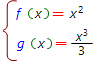# Area between curves online calculator

Plane curves area calculation is one of the main applications of definite integral.To get an area of the plane curve depicted in figure, one needs to calculate definite integral of the form:Functions f(x) and g(x) as a rule are known from a problem situation, abscisses of their cross points x=a and x=b need to be calculated. To do this one need to solve equation of the type:

f(x) = g(x) <=> { x1=a, x2=b }

By using the method above, one can also find the area between f(x) and g(x) disjoint curves, if the points x=a and x=b are initially given:In such a case the crossed curve (figure which area we are calculating) is formed by functions f(x), g(x) and the straight lines x=a, x=b.

Online area calculator based on Wolfram Alpha capable to calculate area between two crossed curves.

## Choose expression input type:

Expression input type:f(x)=
g(x)=